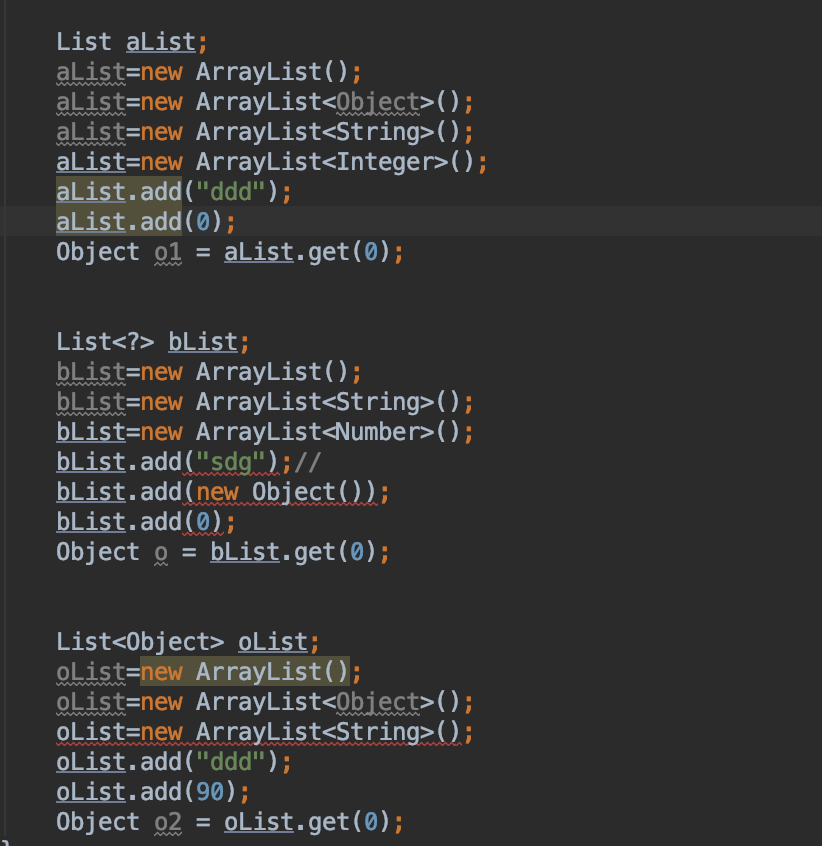## 泛型方法

public <T> String getString(T parm) {
return parm.toString();
}


## 泛型通配符

### <?>

• 只能在声明的时候使用，不能在创建的时候使用， new ArrayList 这样是不可以的，Lit<?> list=null,这样是允许的。
• List<?> 能给他赋值任何类型的 List ，但是不能给他赋值任何类型的数据，因为不知道他存的到底是什么类型。
List<Object> objectList=new ArrayList<String>(); //错误，

List<?> list=new ArrayList<String>(); //正确
//？表示可以是任何类型但是不能确定是什么类型，

//list.add(T t)这个方法的参数是 T ，声明 list 的时候传入的参数化类型是"?",
//而？表示不确定是什么类型，不知道是什么类型所以不能使用这个方法。

Object o = list.get(0);//正确，
//？表示不确定是什么类型，但是所有的类型都继承Object


### 带有下边界的通配符

<? extends Number> ? 只能是 Number 的类或者子类

List<? extends Number> list1;
list1=new ArrayList<Integer>(); //正确 Integer 继承 Number
list1=new ArrayList<Float>();  //正确 Float 继承Number
list1=new ArrayList<String>(); //错误，因为 String 不是 Number 的子类
Number number = list1.get(0); //正确，因为返回的类型肯定是 Number 或者子类，使用父类接收是可以的


### 带有上边界的通配符

<? super Integer> ? 只能是 Number 或者 Number 的父类

List<? super Integer> list2;
list2=new ArrayList<Integer>();//正确
list2=new ArrayList<Number>(); //正确 Number 是父类

list2.add(new Integer(0)); //正确 ， Integer 是子类，

Integer object = list2.get(0); //错误
//因为只能确定返回的是 Integer 或者父类，但是不能确定是哪个父类，所以使用 Integer 接受就错误，只能使用 Object 接受

Object object1 = list2.get(0); //正确


### PECS原则总结

• 如果要从集合中读取类型 T 的数据，并且不能写入，可以使用 ? extends 通配符；(Producer Extends)
• 如果要从集合中写入类型 T 的数据，并且不需要读取，可以使用 ? super 通配符；(Consumer Super)
• 如果既要存又要取，那么就不要使用任何通配符。

## 泛型擦除

java 中的泛型只存在编译时期，运行时， java 虚拟机并不知道泛型的存在，那么带来的问题就是，多态失效

  public void test(List<Integer> value){

}

public void test(List<String> value){

}


## 常见问题

### List ,List<Objec> ,List<?> 的区别

• List<Objec> 的引用只能指向 List 对象，不能指向除了 Object 类型之外的其他对象，而List<?> 和 List 可以
• List 和List<Objec>中可以添加任何类型的对象，因为所有的类型都是 Object 的子类，但是List<?> 不可以，虽然他可以指向任何类型的对象，但是不能确定到底是哪种类型，所以不能往里面添加任何对象，但是可以往从里面取对象，取出来的对象都是 Object 类型
• 编译器不会对 List 进行检查，但是会对List<?> 和List<Objec>进行类型检查 看下图，就能清楚看到### ？和 T 的区别

？代表的是通配符，代表未知类型， T 代表自定义类型，指一种特定的类型

### Class <T extends Comparable<? super T> > 意思

Class 中的类型是 Comparable 或者 Comparable 的子类 T Comparable 中是 T 或者 T 的父类

class Apple implements Comparable<Apple> {
public int weight;

@Override
public int compareTo(Apple o) {
return weight - o.weight;
}
}

class RedApple extends Apple  {}

class MyData<T extends Comparable<T>> {}

public void test() {
MyData<Apple> data = null;// ok
MyData<RedApple> data1 = null; //编译失败
}


MyData<RedApple extends Comparable>

class RedApple extends Apple  {
@Override
public int compareTo(Apple o) { //类型是 Apple 而不是RedApple
return super.compareTo(o);
}
}


### 为啥不能创建泛型数组

String[] strings = new String; // 正常
List[] arr0 = new ArrayList; //正常

List<String>[] arr=new ArrayList<String>;//错误，

List<String>[] arr1 = (ArrayList<String>[]) new ArrayList; //正确，
//通过强转，绕过编译器实例化检查

arr1=new ArrayList<String>();//正确
arr1=new ArrayList<Integer>(); //错误，
//类型不合法，因为通过强转， java 已经获悉这个数组在运行时要应持有的具体数据类型，


public static  <T> T[] creatArr(T[] arg) {
return arg;
}

public static void main(String[] args) {
Integer[] arr={1, 2 , 3 ,4};
String[] arrStr={"a","b","C"};
System.out.printf(Arrays.toString(creatArr(arr)));
System.out.printf(Arrays.toString(creatArr(arrStr)));
}


public static <T> T[] creatArr(int size) {
return (T[]) new Object[size];
}